Latest Banking jobs   »   Quantitative Aptitude Quiz For IBPS Clerk...

# Quantitative Aptitude Quiz For IBPS Clerk Prelims 2021-16th December

Directions (1-5): In each of these questions, two equations (I) and (II) are given. You have to solve both the equations and give answer.
(a) if x>y
(b) if x≥y
(c) if x<y
(d) if x ≤y
(e) if x = y or no relation can be established between x and y.

Q1. I. 6x² + x – 15 = 0
II. 4y² – 24y + 35 = 0

Q2. I. 5x² + 11x + 2 = 0
II. 6y² + 11y + 4 = 0

Q3. I. 2x² – 23x + 56 = 0
II. 3y² – 14y + 15 = 0

Q4. I. 7x + 2y = 12
II. 4x + 3y = 5

Q5. I. x² + 13x + 40 = 0
II. y² + 17y + 72 = 0

Directions (6-10): Solve the following equations and mark the correct option given below.
(a) if x>y
(b) if x≥y
(c) if y>x
(d) if y≥x
(e) if x=y or no relation can be established

Q6. I. 6x² + 7x + 2 = 0
II. 3y² + 8y + 5 = 0

Q7. I. 7x² – 23x + 6 = 0
II. y² – 7y + 12 = 0

Q8. I. 5x² + 13x – 6 = 0
II. 2y² + 13y – 7 = 0

Q9. I. 4x + 3y = 4
II. 6x + 5y = 8

Q10. I. x² – 19x + 88 = 0
II. y² + y – 56 = 0

Directions (11-15): In each question two equations numbered (I) and (II) are given. You should solve both the equations and mark appropriate answer.
(a) If x=y or no relation can be established
(b) If x>y
(c) If x<y
(d) If x≥y
(e) If x≤y

Q11. I. 15x² + 38x + 16 = 0
II. 8y² + 20y + 12 = 0

Q12. I. 8x² + 19x + 11 = 0
II. 8y² + 27y + 22 = 0

Q13. I. 6x² – 9x + 3 = 0
II. 8y² – 11y + 3 = 0

Q14. I. 5x² + 6x – 11 = 0
II. 20y² + 23y – 43 = 0

Q15. I. 3x + 7y = 28
II. 5x + 3y = 38

Solutions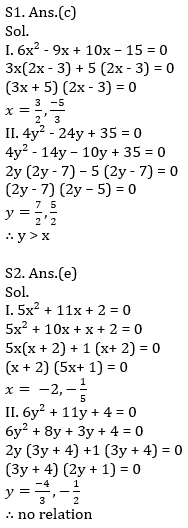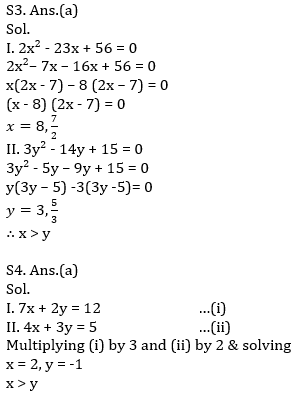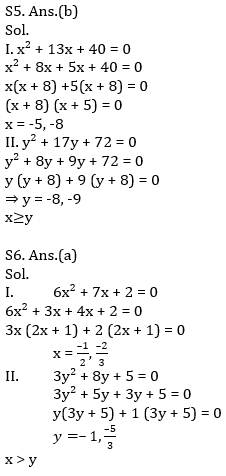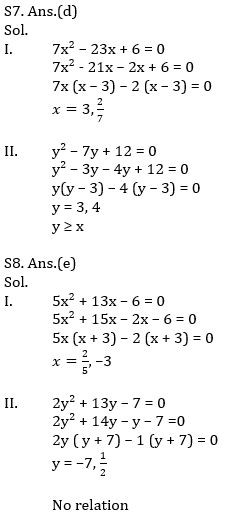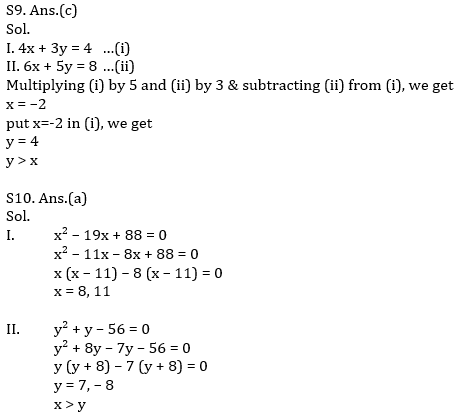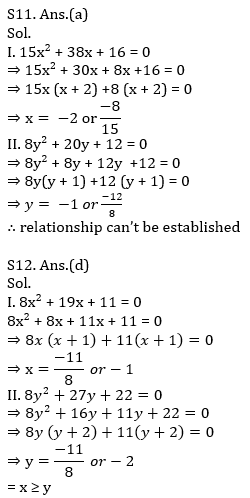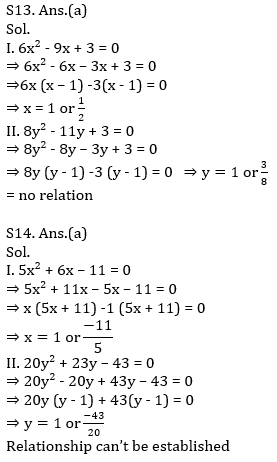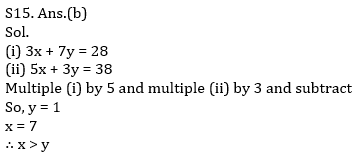Click Here to Register for Bank Exams 2021 Preparation Material#### Congratulations!Download Hindu Review of October 2021: Free PDF